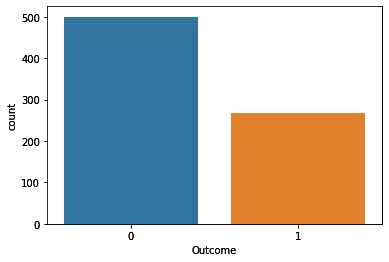• +91-9872993883
• +91-8283824812
• info@ris-ai.com

# Predict Diabetes with Machine Learning ¶

Diabetes is among critical diseases and lots of people are suffering from this disease. Age, obesity, lack of exercise, hereditary diabetes, living style, bad diet, high blood pressure, etc. can cause Diabetes Mellitus. People having diabetes have high risk of diseases like heart disease, kidney disease, stroke, eye problem, nerve damage, etc.

In this article, I will show you how you can use machine learning to Predict Diabetes using Python.

Now let’s import the data and gets started:

In :
import pandas as pd
import numpy as np
import matplotlib.pyplot as plt
%matplotlib inline
print(diabetes.columns)

Index(['Pregnancies', 'Glucose', 'BloodPressure', 'SkinThickness', 'Insulin',
'BMI', 'DiabetesPedigreeFunction', 'Age', 'Outcome'],
dtype='object')

In :
diabetes.head()

Out:
Pregnancies Glucose BloodPressure SkinThickness Insulin BMI DiabetesPedigreeFunction Age Outcome
0 6 148 72 35 0 33.6 0.627 50 1
1 1 85 66 29 0 26.6 0.351 31 0
2 8 183 64 0 0 23.3 0.672 32 1
3 1 89 66 23 94 28.1 0.167 21 0
4 0 137 40 35 168 43.1 2.288 33 1

The diabetes data set consists of 768 data points, with 9 features each:

In :
print("dimension of diabetes data: {}".format(diabetes.shape))

dimension of diabetes data: (768, 9)


“Outcome” is the feature we are going to predict, 0 means No diabetes, 1 means diabetes. Of these 768 data points, 500 are labeled as 0 and 268 as 1:

In :
print(diabetes.groupby('Outcome').size())

Outcome
0    500
1    268
dtype: int64

/usr/local/lib/python3.6/dist-packages/seaborn/_decorators.py:43: FutureWarning: Pass the following variable as a keyword arg: x. From version 0.12, the only valid positional argument will be data, and passing other arguments without an explicit keyword will result in an error or misinterpretation.
FutureWarning

In :
import seaborn as sns
sns.countplot(diabetes['Outcome'],label="Count")

Out:
<AxesSubplot:xlabel='Outcome', ylabel='count'>In :
diabetes.info()

<class 'pandas.core.frame.DataFrame'>
RangeIndex: 768 entries, 0 to 767
Data columns (total 9 columns):
#   Column                    Non-Null Count  Dtype
---  ------                    --------------  -----
0   Pregnancies               768 non-null    int64
1   Glucose                   768 non-null    int64
2   BloodPressure             768 non-null    int64
3   SkinThickness             768 non-null    int64
4   Insulin                   768 non-null    int64
5   BMI                       768 non-null    float64
6   DiabetesPedigreeFunction  768 non-null    float64
7   Age                       768 non-null    int64
8   Outcome                   768 non-null    int64
dtypes: float64(2), int64(7)
memory usage: 54.1 KB


### K-Nearest Neighbors to Predict Diabetes ¶

The k-Nearest Neighbors algorithm is arguably the simplest machine learning algorithm. Building the model consists only of storing the training data set. To make a prediction for a new point in the dataset, the algorithm finds the closest data points in the training data set — its “nearest neighbors.”

First, Let’s investigate whether we can confirm the connection between model complexity and accuracy:

In :
from sklearn.model_selection import train_test_split
X_train, X_test, y_train, y_test = train_test_split(diabetes.loc[:, diabetes.columns != 'Outcome'], diabetes['Outcome'], stratify=diabetes['Outcome'], random_state=66)
from sklearn.neighbors import KNeighborsClassifier
training_accuracy = []
test_accuracy = []
# try n_neighbors from 1 to 10
neighbors_settings = range(1, 11)
for n_neighbors in neighbors_settings:
# build the model
knn = KNeighborsClassifier(n_neighbors=n_neighbors)
knn.fit(X_train, y_train)
# record training set accuracy
training_accuracy.append(knn.score(X_train, y_train))
# record test set accuracy
test_accuracy.append(knn.score(X_test, y_test))
plt.plot(neighbors_settings, training_accuracy, label="training accuracy")
plt.plot(neighbors_settings, test_accuracy, label="test accuracy")
plt.ylabel("Accuracy")
plt.xlabel("n_neighbors")
plt.legend()

Out:
<matplotlib.legend.Legend at 0x7f0923026518>Let’s check the accuracy score of the k-nearest neighbors algorithm to predict diabetes.

In :
knn = KNeighborsClassifier(n_neighbors=9)
knn.fit(X_train, y_train)
print('Accuracy of K-NN classifier on training set: {:.2f}'.format(knn.score(X_train, y_train)))
print('Accuracy of K-NN classifier on test set: {:.2f}'.format(knn.score(X_test, y_test)))

Accuracy of K-NN classifier on training set: 0.79
Accuracy of K-NN classifier on test set: 0.78


Now, Let's predict for a random array having the value for independent features say Xn:

In :
Xn = [[5, 108, 72, 43, 75,36.1, 0.263, 33]]
out = knn.predict(Xn)
if out == 0:
print("No Diabetes")
else:
print("Diabetes")

No Diabetes


From the above we see that person with 'Xn' values has no diabetes. I hope you liked this article to predict diabetes with Machine Learning.